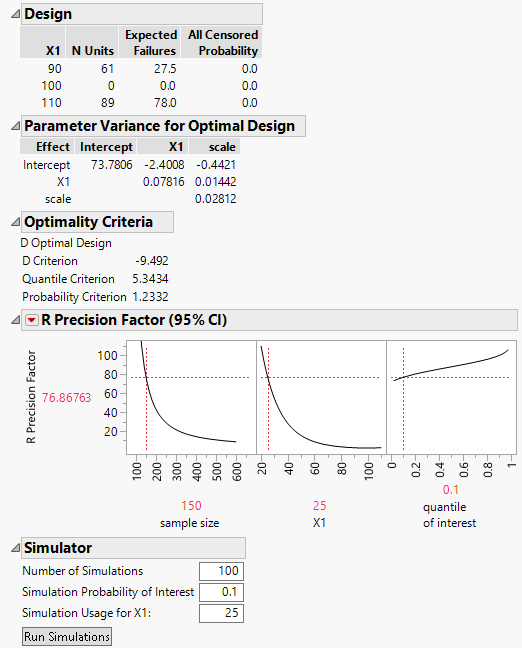Publication date: 08/13/2020

## Create and Assess the Optimal Design

Click Make Design to create the optimal design and see results that address the quality of the design. By default, the optimal design is D-optimal. You can change the optimality criterion by selecting the red triangle option ALT Optimality Criterion.

When you click Make Design, the following report appears:

Figure 22.20 Design OutlinesDesign

Shows the number of units to be tested at each combination of acceleration levels. For a single factor, the first column gives the levels of the factor. For two factors, the first two columns give the levels of the factors.

N Units

The number of units to be tested at each combination of acceleration levels.

Expected Failures

Expected number of failures for the design setting. The expected number is computed using the prior model specification.

All Censored Probability

Probability that none of the units tested at the combination of acceleration levels fail. The probability is computed using the prior model specification.

Note: The expected failures and censoring probabilities enable you to judge whether your prior specifications are reasonable.

Parameter Variance for Optimal Design

Shows a matrix proportional to the covariance matrix for the estimates of the acceleration model parameters for the optimal design. The calculation assumes that the values specified in the Prior Mean outline are the true parameter values. See Parameter Variance for Balanced Design.

Note: Compare the values in the Parameter Variance for Optimal Design matrix to those in the Parameter Variance for Balanced Design matrix to determine the extent to which the optimal design reduces the variance of estimates.

Optimality Criteria

Values for three optimality criteria appear:

D Criterion

D-optimality of the design. See Make D-Optimal Design.

Quantile Criterion

Time I-optimality of the design. See Make Quantile Estimate Optimal.

Probability Criterion

Probability I-optimality of the design. See Make Failure Probability Optimal.

R Precision Factor (95% CI)

A profiler to evaluate the impact of sample size, level of the factor(s), and the quantile of interest on the R Precision Factor. The R Precision Factor (95% CI) reflects the confidence interval for the quantile of interest.

Simulator

Use the simulator to explore your accelerated model before collecting data.

Number of Simulations

The number of simulation trials to run.

Simulation Probability of Interest

The failure probability of interest.

Simulation Usage

Values for the usage conditions of the acceleration factors.

After clicking Run Simulations, a table of summary statistics and a plot of model fits appear.